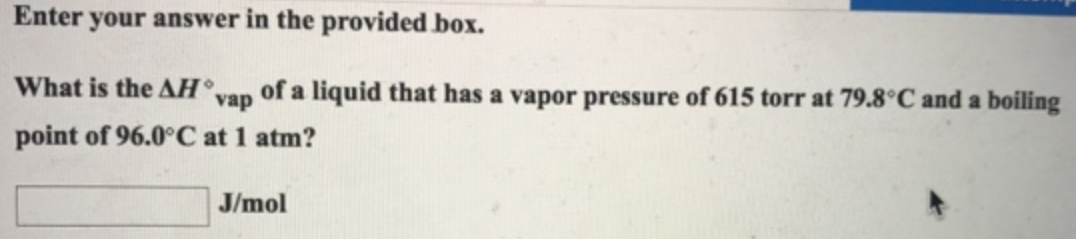Chemistry Practice Problems Clausius-Clapeyron Equation Practice Problems Solution: Enter your answer in the provided box. What is the...

# Solution: Enter your answer in the provided box. What is the ΔH°vap of a liquid that has a vapor pressure of 615 torr at 79.8 °C and a boiling point of 96.0 °C at 1 atm?

###### Problem

What is the ΔH°vap of a liquid that has a vapor pressure of 615 torr at 79.8 °C and a boiling point of 96.0 °C at 1 atm?View Complete Written Solution

Clausius-Clapeyron Equation

Clausius-Clapeyron Equation

#### Q. A certain liquid has a vapor pressure of 92.0 Torr at 23.0°C and 290.0 Torr at 45.0°C. Calculate the value of ΔH°vap for this liquid.

Solved • Wed Jul 25 2018 14:53:49 GMT-0400 (EDT)

Clausius-Clapeyron Equation

#### Q. For a substance with a molar enthalpy of vaporization of 83 kJ/mol, how much must the temperature be lowered from 600 K to decrease the vapor pressure...

Solved • Tue Jul 24 2018 11:31:50 GMT-0400 (EDT)

Clausius-Clapeyron Equation

#### Q. A certain liquid has a vapor pressure of 92.0 Torr at 23.0°C and 203.0 Torr at 45.0°C. Calculate the value of ΔH°vap for this liquid. Calculate the no...

Solved • Mon Jul 16 2018 13:07:17 GMT-0400 (EDT)

Clausius-Clapeyron Equation

#### Q. A certain substance has a heat of vaporization of 43.09 kJ/mol. At what Kelvin temperature will the vapor pressure be 3.00 times higher than it was at...

Solved • Tue Jul 03 2018 11:37:20 GMT-0400 (EDT)